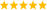# LabVIEW

cancel
Showing results for
Did you mean:

## integfration function

Hi every one,

Is there any way to get the definite integration of a function? I need to do some calculations in my code which one of these is like "Integration of EXP(-x^2)dx from x=-inf to x=+inf". Can anyone please help me?

Thanks

Message 1 of 5
(924 Views)

## Re: integfration function

Hi Soran,

running a loop from -INF to +INF with a small dx can take quite a long time. 🙂

Hint: You should rather use MATLAB or MATHEMATICA for such equations. Or paper and pencil…

Best regards,
GerdW
CLAD expired, using LV2011SP1 + LV2017 (+LV2019 sometimes) on Win7+cRIO
Kudos are welcomeMessage 2 of 5
(900 Views)

## Re: integfration function

LabVIEW does not do symbolic math. For questions like this, ask wolfram alpha, it even understands your question as written.The answer is sqrt(pi), of course.LabVIEW Champion. It all comes together inGCentralMessage 3 of 5
(893 Views)

## Re: integfration function

Actually I do not think I can use wolfram (or paper and pencil!) to do this. The integration I mentioned above was just an example of what I should do. In fact, it will be something like this: "EXP(-x^2)/(a^2+(b-x)^2) x=-inf to x=+inf" in which a and b are two constants (relative to x) related to pressure and temperature (which change continuously). So some parts of my code read the temperature and pressure and then should substitute their values inside this formula and finally get the tesult of the integral.

Thanks,

Soran

Message 4 of 5
(863 Views)

## Re: integfration function

You simply use wolfram alpha again (or any other symbolic math tool) and it will give you the resulting formula in terms of a and b (need to upgrade to pro, though). Then program it in LabVIEW.LabVIEW Champion. It all comes together inGCentralMessage 5 of 5
(859 Views)